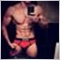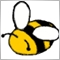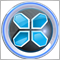# MQL4 iCustom / I do not receive the right value29

I looked for the solution for a while, but nothing was right for me. Ill attach an Indicator. The problem is that iCustom return Empity Value (2147483647).

That-s my simple code:

```//+------------------------------------------------------------------+
//|                                              BeaterFX_MTF+PA.mq4 |
//|                        Copyright 2019, MetaQuotes Software Corp. |
//|                                             https://www.mql5.com |
//+------------------------------------------------------------------+
#property version   "1.00"
#property strict

static datetime lastbar;
double K_MTF,D_MTF,K_MTF2,D_MTF2,mySTO;
//+------------------------------------------------------------------+
//| Expert initialization function                                   |
//+------------------------------------------------------------------+
int OnInit()
{
//---
lastbar=0;
//---
return(INIT_SUCCEEDED);
}
//+------------------------------------------------------------------+
//| Expert deinitialization function                                 |
//+------------------------------------------------------------------+
void OnDeinit(const int reason)
{
//---

}
//+------------------------------------------------------------------+
//| Expert tick function                                             |
//+------------------------------------------------------------------+
void OnTick()
{
//---

K_MTF = iCustom(NULL,PERIOD_H1,"MTF Stochastic v21",1,1);
D_MTF = iCustom(NULL,PERIOD_H1,"MTF Stochastic v21",1,1);
K_MTF2 = iCustom(NULL,PERIOD_H1,"MTF Stochastic v21",1,2);
D_MTF2 = iCustom(NULL,PERIOD_H1,"MTF Stochastic v21",2,2);

if(isNewBar())
{
checkForEntry();
}
}
//+------------------------------------------------------------------+

void checkForEntry()
{
if(D_MTF>=45 && K_MTF>50 && D_MTF2<45)
{
Print("DMTF/KMTF: "+NormalizeDouble(D_MTF,2)+" - "+NormalizeDouble(K_MTF,2));
}
if(D_MTF<=55 && K_MTF<50 && D_MTF2>55)
{
OrderSend(Symbol(),ORDER_TYPE_SELL,0.5,Bid,3,0,0,"SELL",2222,0,clrOrange);
}

if(OrdersTotal()>0 && ((K_MTF>D_MTF && K_MTF2<=D_MTF2) || (K_MTF<D_MTF && K_MTF2>=D_MTF2)))
{
CloseAll();
}
}
//+------------------------------------------------------------------+

void CloseAll()
{
int numOfOrders=OrdersTotal();
int FirstOrderType=0;

for(int index=0; index<OrdersTotal(); index++)
{
if(OrderSymbol()==Symbol())
{
FirstOrderType=OrderType();
break;
}
}

for(int index=numOfOrders-1; index>=0; index--)
{

if(OrderSymbol()==Symbol())
switch(OrderType())
{
if(!OrderClose(OrderTicket(),OrderLots(),MarketInfo(OrderSymbol(),MODE_BID),3,clrRed))
break;

case OP_SELL:
break;

case OP_SELLLIMIT:
case OP_SELLSTOP:
if(!OrderDelete(OrderTicket()))
break;
}
}
}
//+------------------------------------------------------------------+

bool isNewBar()
{
datetime curbar=Time;

if(lastbar!=curbar)
{
lastbar=curbar;
return (true);
}

else
{
return(false);
}

}
//+------------------------------------------------------------------+```
Files:489

BTW, I don't think Empty_Value is equal to INT_MAX !!!29403

Hi,

So you would get data from buffer 0 & 1,others dont have any value:

```   K_MTF_1 = iCustom(_Symbol,PERIOD_H1,"MTF Stochastic v21",0,1);
D_MTF_1 = iCustom(_Symbol,PERIOD_H1,"MTF Stochastic v21",1,1);

K_MTF_2 = iCustom(_Symbol,PERIOD_H1,"MTF Stochastic v21",0,2);
D_MTF_2 = iCustom(_Symbol,PERIOD_H1,"MTF Stochastic v21",1,2);```

Regards.1351

Luca Minoi: